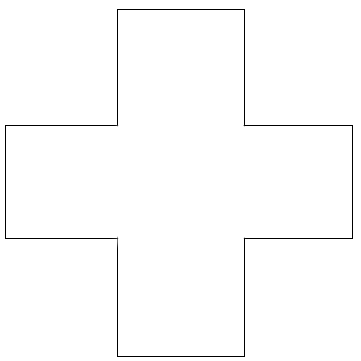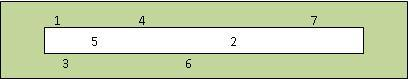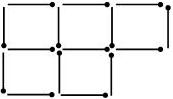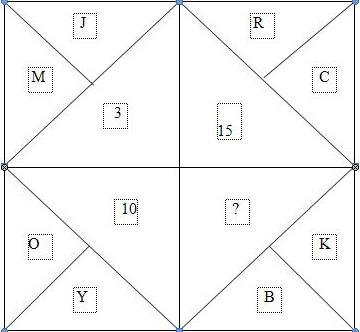# Medium Logical Puzzles - 1

Logical Reasoning Puzzles form an essential part of bank PO, SSC, government exams and many entrance tests. These are mostly arrangement-based or picture puzzles. Such questions intend to check your analytical, deductive and problem solving abilities. Here, we have compiled a series of different logical puzzles with solutions. This set contains 10 medium level logic puzzles.
Solve the given questions and check your logical abilities:
Q.1. The letters J, K, L, M, N, O and Z, represent seven consecutive integers from 42 to 53; not necessarily in that order,
O is as much less than K as L is greater than M.
Z is greater than O.
K is the middle term.
J is 3 greater than M.
Can you find the sequence of letters from the lowest value to the highest value?
Q.2. There are 3 points labeled X, Y and Z. Then there are other 3 points labeled 5, 6 and 7. The aim of the puzzle is to connect point X with points 5, 6 and 7, point Y with points 5, 6 and 7 and point Z with points 5, 6 and 7. While connecting the points you have to follow one rule - the lines cannot cross over each other.
 X Y Z 5 6 7
You can arrange the points in order as long as the lines DO NOT cross over each other.
Q.3. A RAW agent code named Chandrakanta emailed a code word to his head office. They are "AIS EUC RAO REI COS". But four of these five words are fake and only one contains the information.
He also mailed a sentence as a clue - if I tell you any one character of the code word, you would be able to tell the number of vowels in the code word. Can you tell what the code word is?
Q.4. At a farewell party, people were asked to guess how many marbles  there were in a box.
No one guessed correctly, but the nearest guesses were 171, 177, 186 and 190.
The correct number of marbles was one unit away from one of the closest guess, three units away from another, ten units away from the third and sixteen units away from the remaining closest guess. How many marbles were there in the box?
Q.5. Here is an ordinary cross.
You are allowed to make two straight cuts across it.
How do you cut it to make the most pieces?Q.6. Digits 1 through 7 are printed above the box, in the box, and beneath the box. Can you continue on for 8 & 9 and place them correctly according to the given scheme?Q.7. 15 matches made 5 squares as shown in the figure.One has to move 3 matches in order to make 4 squares.Q.8.Find the missing character from the given alternatives.1. 5
2. 9
3. 11
4. 13
Q.9. J x K means that J is the mother of K, J + K means that J is the father of K, J - K means that J is the sister of K. On the basis of this information, you have to select the option which shows that L is the grandfather of K.
1. L + J +M – K
2. L x M + N – K
3. L + N - K
4. L +M - N x K
5. L – N x K
Q.10. There are 70 teachers employed with hitbullseye company, of which 30 are females.
(1) 30 teachers are married.
(2) 24 teachers are above 31 years of age.
(3) 19 married teachers are above 31 years of which 7 are males.
(4) 12 males are above 31 years of age.
(5) 15 males are married.
How many of unmarried females are above 31 years of age?